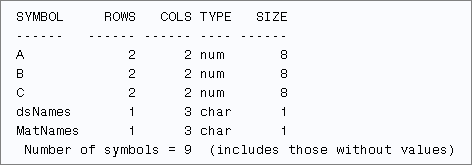Do you have dozens (or even hundreds) of SAS data sets that you want to read into SAS/IML matrices? In a previous blog post, I showed how to iterate over a series of data sets and analyze each one. Inside the loop, I read each data set into a matrix X. I computed some quantity, and then reused X for the next data set. This technique works well, but it assumes that you only need to analyze one matrix at a time. How can you read the data sets into different matrices so that all the matrices reside in memory concurrently?

The trick is to use the SAS/IML VALSET routine to assign the contents of each data set to a unique matrix name. As I showed in a previous article, the VALSET call can dynamically create matrices whose names are specified at run time. This enables you to automate the reading of data sets into SAS/IML matrices.

The following program creates three data sets and reads the data into SAS/IML matrices whose names are identical to the data set names:

```/* create square matrices in data sets */ data A; x=1; y=2; output; x=2; y=3; output; run; data B; set A; x = x + 2; run; data C; set A; y = y + 1; run;   /* read data into SAS/IML matrices of the same name */ proc iml; dsNames = {A B C}; /* specify names of data set */ MatNames = dsNames; /* specify names of matrices */ do i = 1 to ncol(dsNames); use (dsNames[i]); read all var _NUM_ into X; /* read data into X */ call valset(MatNames[i], X); /* copy data to matrix with specified name */ close (dsNames[i]); end; free i X; show names;```The READ statement reads all numerical data into the matrix X. The VALSET call copies data from X into a matrix whose name is stored in MatNames[i]. The SHOW NAMES statement displays the names of all matrices that have values. From the output, you can see that the program creates matrices named A, B, and C.

For this example, the names of the matrices are the same as the names of the data sets. Of course, the matrices could have different names. You can assign MatName to be any array of valid names. For example, the following statement specifies the matrix names as X1, X2, and X3:

`MatNames = "x1":("x"+strip(char(ncol(dsNames))));`

You can use these ideas to read hundreds of data sets into SAS/IML matrices. For example, suppose that you need to create SAS/IML matrices for all of the numerical data in a library. You can use the DATASETS function to obtain the names of all data sets in a library. There are two special cases that you potentially need to handle:

• Some data sets might not have any numeric variables. To protect against that case, use the _ALL_ keyword to read the data. If a data set contains even one numerical variable, it will be read into the X matrix. If not, the matrix X will be a character matrix, and you can use the TYPE function to check whether X is numeric before you call the VALSET subroutine.
• Some data sets might be empty. To protect against this case, use the FREE statement to free X matrix you read each data set. If the next data set is empty, then the X matrix will be undefined. Again, the TYPE function can check for this situation.

The following SAS/IML program reads all of the data sets in the SASHELP library. On my version of SAS, there are 129 data sets in the library. Two are empty and 10 others do not contain any numerical variables. Consequently, the program defines 117 numerical matrices:

```proc iml; libref = "Sashelp"; /* read from this library */ dsNames = T( datasets(libref) ); /* 129 data sets */ MatNames = dsNames; /* names of matrices */ do i = 1 to ncol(dsNames); ds = libref + "." + strip(dsNames[i]); use (ds); /* open sashelp.filename */ read all var _ALL_ into X; /* read data into X */ if type(X)="N" then /* exclude data with zero rows or all char */ call valset(MatNames[i], X); /* copy data to matrix with specified name */ close (ds); free X; /* in case of empty data sets */ end; show names; /* 117 numerical matrices defined */```The SHOW NAMES statement shows the names of a few of the matrices that were created from the numerical data in the Sashelp library. These matrices reside in memory concurrently. This technique would be useful if you have a SAS program that creates a bunch of matrices (as data sets) that you want to multiply together.

Share